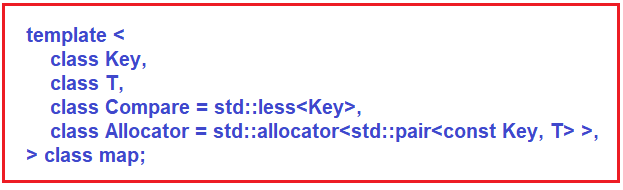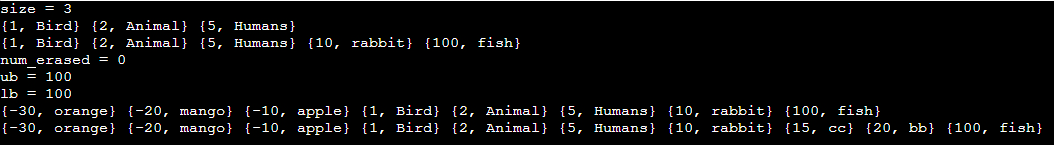# Map in C++

## Map in C++ with Examples:

In this article, I am going to discuss Map in C++ with Examples. Please read our previous article, where we discussed Unordered MultiSet in C++ with Examples.

##### Map in C++ with Examples:

The Map is a very useful data structure and it is an associative container. The Map contains key-value pairs and it is unique. It is a map and not a multi-map so the keys are unique. If you compare it with the set, in the set, we had just one value that acted as a key but here we have a key-value pair i.e. <key, value>. Here the key should be unique but the value can be repeated. So, if <k, v> already exists and we want to insert another pair with the same key with a different value then it will not be inserted. Maps are sorted. Just like a normal set, these are stored in red-black trees.

We have a red-black tree where the root is black or white. Then we can have some children either red or black but no two continuous nodes should be red. These are some of the properties of red-black trees by which it maintains the balance property. The property that helps it is that the black depth of all the leaves is equal and no two continuous nodes can be red. This prevents the tree from being unbalanced. The best case can be that it is perfectly balanced where we have either alternate red-black or all-black nodes. The worst scenario would be that we have alternate red and black. That means the black node will be followed by red followed by black followed by red and so on.

We are trying to increase the left and on the other side the minimum possible would be if we have two black then another side should be a minimum of 2 black. So, this minimum or the closest leaf to the root will be which has all black nodes and the farthest will be which has alternate nodes. Its height will be of the order of log n. This is very similar to the set that we had seen earlier.

For using Map data structure, you need to include the <map> header file. It is sorted just like the normal set but if we see an unordered map, it will not be sorted. It will be implemented using a hash table so it will not be sorted but here it is sorted and you can provide a compare function for comparing the keys. All the operations i.e. search, removal and insertion take logarithmic time because we are using a balanced binary search tree. The height is of the order of log n. It is usually implemented as red-black trees.This is the template where we have a key type comparison. It is std::less so it will be sorted in ascending order. let us see some of the main functionality of the map.

##### Functions of Map in C++:
1. Size () – it will return the number of key-value pairs in the map or the number of nodes in the tree.
2. Equal (=) – you can assign an Initializer list. Here initializer list will be slightly different.
3. List = {{key-value}, {key-value}, … }; This is the initializer list syntax and within this, we will have key-value pairs.
4. [], at () –‘[]’ is used to access the value i.e. you have a map m = {{5, ‘a’}, {7, ‘n’}}; then m  will print ‘a’. ‘at’ function will do the same thing but one thing it will do that is bound checking. If some key is not present in your map, it will throw an exception. Both of these ‘[]’ and ‘at’ can be used to assign value.
5. Clear () – it will remove everything inside the map.
6. Count (), find () – if you write m.count (5), since duplicates are not allowed it will be 0 or 1. If you write m.find (5), it will return the iterator to the pair having the first element as 5. If the given key is not present it will return m.end () which does not point to any of the elements. It is a hypothetic iterator pointing to the one position past the last element.
7. Empty () – it checks whether any key-value pairs are present or not.
8. Begin (), end () – begin returns the beginning of the iterator to the first element or smallest element depending on the comparator. By default, it’s less. ‘end’ return the iterator to the one position past the last element.
9. upper_bound (key), lower_bound (key) –upper bound is more than (>), and lower bound is more than equal (>=). Let’s say we have a list v = {1, 2, 4, 5, 7, 10}. Now if we call these functions as,
10. upper_bound (5); // it will return 10
11. lower_bound (5); // it will return 5

if we call upper_bound (6) which is not present in the list, it will return 7, and lower_bound (6) will also return 7.

##### Insert Function of Map in C++:
1. Insert (p): It inserts a pair i.e. p and it returns a pair. The first value will indicate the iterator to inserted element if the element was inserted otherwise if it is already present then the element will not be inserted. The second value is of bool type which denotes true if insertion takes place and false if the element already exists.
2. Insert (iterator pos1, iterator pos2): Here, we can pass two iterator positions. Let’s say you have a map m with some pairs, m = {_ _ _ x _ _ _ y _ _ _ _ } You want to insert from position x to position y (excluding y) and these are iterator positions. It is like range [pos1, pos2). So, the close interval at pos1 and open interval at pos2. This function will not return anything.
3. Insert (initializer_list l): Similarly, we can pass the initializer list. You can think of it as in the above example if we are inserting from pos1 to pos2, so it will be an initializer list or you can say a subset of the map. So, you will pass that initializer list and it will add all the elements to the map. It will also not return anything. We will see examples of all of these functions.
##### Erase Functions of Map in C++:
1. erase (iterator pos): It removes the element at the given position and returns the iterator following the last element removed.
2. erase (iterator pos1, iterator pos2): As we have seen in the insert function, we can also pass two positions in this function so that all the elements between these positions will be removed. So pos1 will be included and pos2 will be excluded. It also returns the iterator following the last element removed.
3. erase (key): In this signature, we have passed the iterator to the key but here we are passing the key itself. If any key is present in the map, it will remove all the elements with the key values. It will return the number of elements removed.

Now let’s see the complete program.

##### Example to understand the Map in C++:
```#include <iostream>
#include <map>
using namespace std;

int main() {
map<int, string> m = {{1, "Bird"}, {2, "Animal"}, {5, "Humans"}};

cout << "size = " << m.size() << endl;
for(auto& p: m)
cout << "{" << p.first << ", " << p.second << "} ";
cout << endl;

m.insert({10, "rabbit"});
m.insert({100, "fish"});

for(auto& p: m)
cout << "{" << p.first << ", " << p.second << "} ";
cout << endl;

//auto it = m.erase(m.find(10));
//cout << it->first << endl;

int num_erased = m.erase(15);
cout << "num_erased = " << num_erased << endl;

auto ub = m.upper_bound(15);
auto lb = m.lower_bound(15);
cout << "ub = " << ub->first << endl;
cout << "lb = " << lb->first << endl;

m.insert({{-10, "apple"}, {-30, "orange"}, {-20, "mango"}});
for(auto& p: m)
cout << "{" << p.first << ", " << p.second << "} ";
cout << endl;

map<int, string> m2 = {{10, "aa"}, {20, "bb"}, {15, "cc"}, {5, "dd"}};
m.insert(m2.begin(), m2.end());

for(auto& p: m)
cout << "{" << p.first << ", " << p.second << "} ";
cout << endl;

}
```
###### Output: